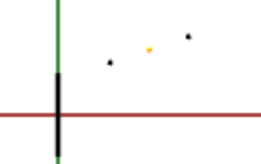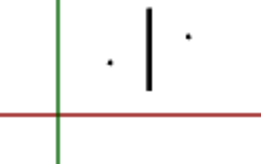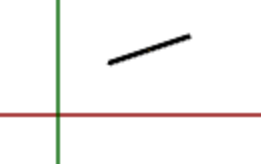by Rahel Lüthy

March 22, 2015

# JavaFX 3D Line

I have no experience in 3D programming whatsoever. Last week, I took my first steps in JavaFX 3D, but hit a wall when I wanted to draw a line between two points: There is no `Line` shape! The only out-of-the-box `Shape3D` classes are `Box`, `Cylinder`, `Sphere`, and `MeshView` – wow…

Well, it shouldn’t be too difficult to connect the points with a slender cylinder, right?

I was wrong, it took me an embarrassingly long time to get the trigonometry right :-)

Here’s my magic crutch:

``````public Cylinder createConnection(Point3D origin, Point3D target) {
Point3D yAxis = new Point3D(0, 1, 0);
Point3D diff = target.subtract(origin);
double height = diff.magnitude();

Point3D mid = target.midpoint(origin);
Translate moveToMidpoint = new Translate(mid.getX(), mid.getY(), mid.getZ());

Point3D axisOfRotation = diff.crossProduct(yAxis);
double angle = Math.acos(diff.normalize().dotProduct(yAxis));
Rotate rotateAroundCenter = new Rotate(-Math.toDegrees(angle), axisOfRotation);

Cylinder line = new Cylinder(1, height);

return line;
}``````

To illustrate what’s going on, I created a 2D representation of all individual steps, starting off with the creation of the cylinder. By default, its center is placed at the origin:The `moveToMidpoint` transformation moves its center to the final location (the yellow midpoint in the above image):And finally, the `rotateAroundCenter` transformation corrects the cylinder’s direction:Obviously, the tricky part is finding the proper axis and angle of rotation. Both are calculated relative to the `yAxis` because of the cylinder’s initial direction. The `axisOfRotation` must be perpendicular to the plane defined by the `yAxis` and the `diff` vector. To find such a perpendicular vector, we can (by definition) calculate the cross product of the two vectors. In the 2D case, the result is equivalent to the z-axis, but in 3D it may be tilted.

The angle is calculated based on the dot product (aka scalar product) of the two vectors, which is defined by

`A • B = |A| |B| cos(alpha)`

If `A` and `B` are both unit vectors, this becomes

`A • B = cos(alpha)`

which we can resolve to

`alpha = acos(A • B)`

That’s it!